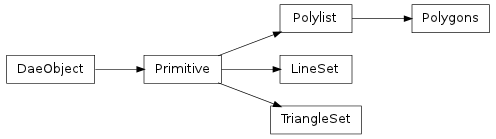#### Next topic

class collada.polylist.Polylist(sources, material, index, vcounts, xmlnode=None)

Class containing the data COLLADA puts in a <polylist> tag, a collection of polygons. The Polylist object is read-only. To modify a Polylist, create a new instance using collada.geometry.Geometry.createPolylist().

• If P is an instance of collada.polylist.Polylist, then len(P) returns the number of polygons in the set. P[i] returns the ith polygon in the set.__init__(sources, material, index, vcounts, xmlnode=None)

A Polylist should not be created manually. Instead, call the collada.geometry.Geometry.createPolylist() method after creating a geometry instance.

Methods

 __init__(sources, material, index, vcounts) A Polylist should not be created manually. Instead, call the bind(matrix, materialnodebysymbol) Create a bound polylist from this polylist, transform and material mapping load(collada, localscope, node) save() triangleset() This performs a simple triangulation of the polylist using the fanning method.

Attributes

 normal Read-only numpy.array of size Nx3 where N is the number of normal values in the normal_index Read-only numpy.array of size Nx3 where N is the number of vertices in the primitive. texcoord_indexset Read-only tuple of texture coordinate index arrays. texcoordset Read-only tuple of texture coordinate arrays. vertex Read-only numpy.array of size Nx3 where N is the number of vertex points in the vertex_index Read-only numpy.array of size Nx3 where N is the number of vertices in the primitive.
xmlnode

ElementTree representation of the line set.

triangleset()

This performs a simple triangulation of the polylist using the fanning method.

bind(matrix, materialnodebysymbol)

Create a bound polylist from this polylist, transform and material mapping

getInputList()

Gets a collada.source.InputList representing the inputs from a primitive

normal

Read-only numpy.array of size Nx3 where N is the number of normal values in the primitive’s normal source array.

normal_index

Read-only numpy.array of size Nx3 where N is the number of vertices in the primitive. To get the actual normal values, one can use this array to select into the normals array, e.g. normal[normal_index].

texbinormal_indexset

Read-only tuple of texture binormal index arrays. Each value is a numpy.array of size Nx3 where N is the number of vertices in the primitive. To get the actual texture binormals, one can use the array to select into the texbinormalset array, e.g. texbinormalset[texbinormal_indexset] would select the first set of texture binormals.

texbinormalset

Read-only tuple of texture binormal arrays. Each value is a numpy.array of size Nx3 where N is the number of texture binormals in the primitive’s source array.

texcoord_indexset

Read-only tuple of texture coordinate index arrays. Each value is a numpy.array of size Nx2 where N is the number of vertices in the primitive. To get the actual texture coordinates, one can use the array to select into the texcoordset array, e.g. texcoordset[texcoord_indexset] would select the first set of texture coordinates.

texcoordset

Read-only tuple of texture coordinate arrays. Each value is a numpy.array of size Nx2 where N is the number of texture coordinates in the primitive’s source array.

textangent_indexset

Read-only tuple of texture tangent index arrays. Each value is a numpy.array of size Nx3 where N is the number of vertices in the primitive. To get the actual texture tangents, one can use the array to select into the textangentset array, e.g. textangentset[textangent_indexset] would select the first set of texture tangents.

textangentset

Read-only tuple of texture tangent arrays. Each value is a numpy.array of size Nx3 where N is the number of texture tangents in the primitive’s source array.

vertex

Read-only numpy.array of size Nx3 where N is the number of vertex points in the primitive’s vertex source array.

vertex_index

Read-only numpy.array of size Nx3 where N is the number of vertices in the primitive. To get the actual vertex points, one can use this array to select into the vertex array, e.g. vertex[vertex_index].